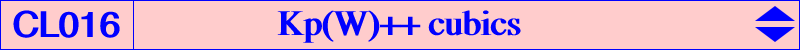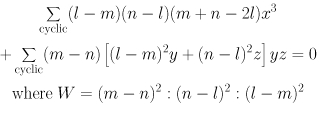The cubics Kp(W) and Kc(W) are K++ cubics if and only if the pole W of the isoconjugation lies on the inscribed Steiner ellipse. Hence, one of the fixed points of the isoconjugation, say F, lies on the line at infinity, being the infinite point of a line L passing through G. The others are called Ra, Rb, Rc. W is the complement of the isotomic conjugate of the infinite point of this line. For any such point W, Kc(W) is now denoted Kc(W)++ and decomposes into L and a diagonal parabola inscribed in the medial triangle. This parabola is the complement of another parabola inscribed in ABC, with perspector the isotomic conjugate of F, with focus the isogonal conjugate of F, with directrix the perpendicular at H to L. Kp(W) becomes Kp(W)++ and is now a Newton trident with a triple point at infinity which is F. K079 is the special case where W = X(115). For any point (l : m : n) distinct of G, the barycentric equation of Kp(W)++ is :